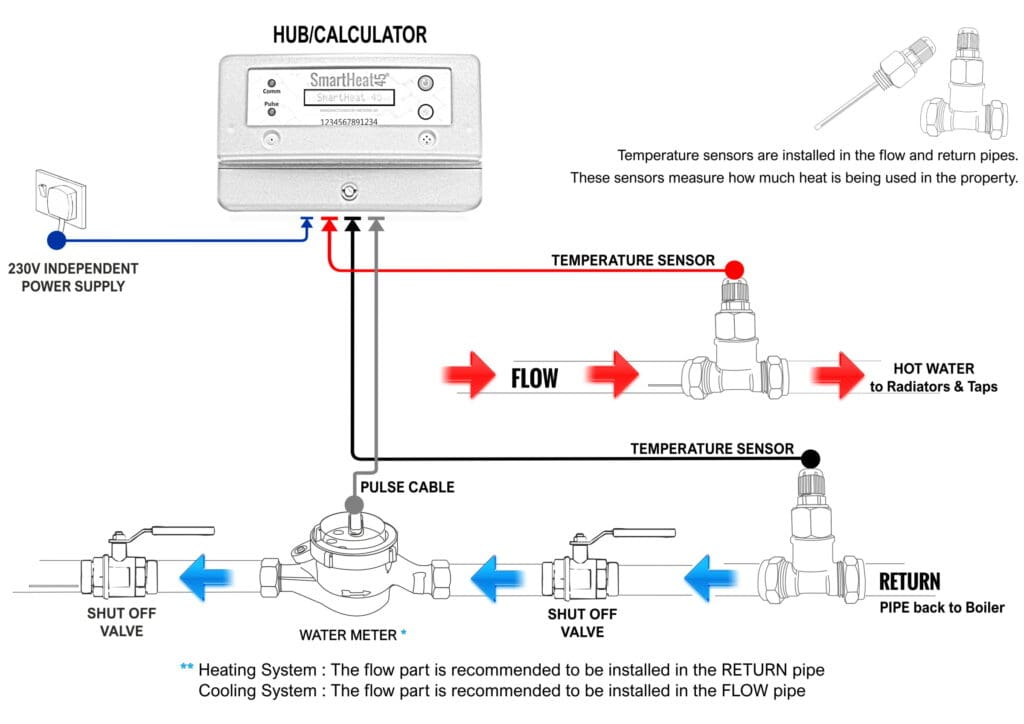The Heat meter calculates the difference between the energy in the hot water and the energy in the returning cold water.  This energy difference is then multiplied by the density and flow to give the total power used.

<Back